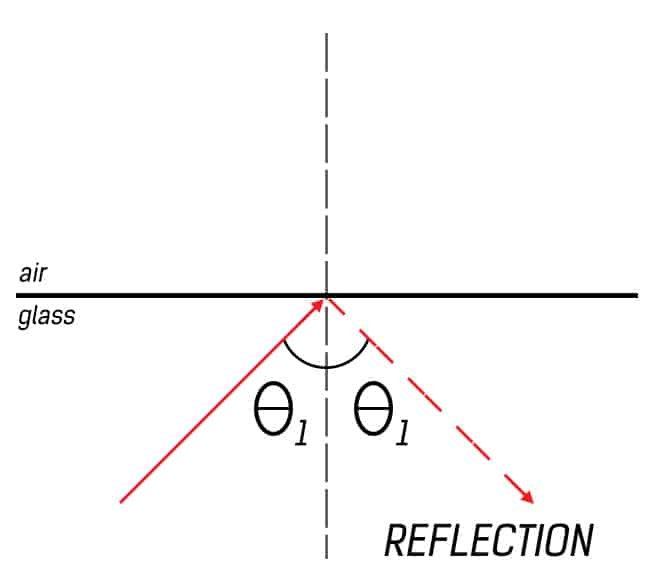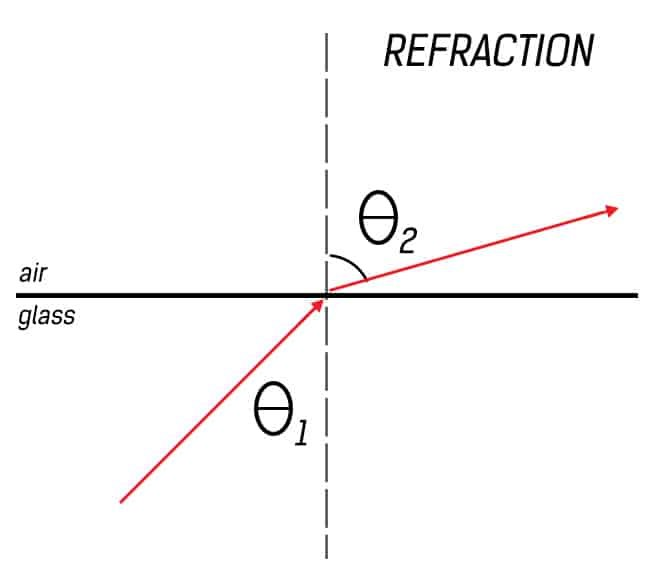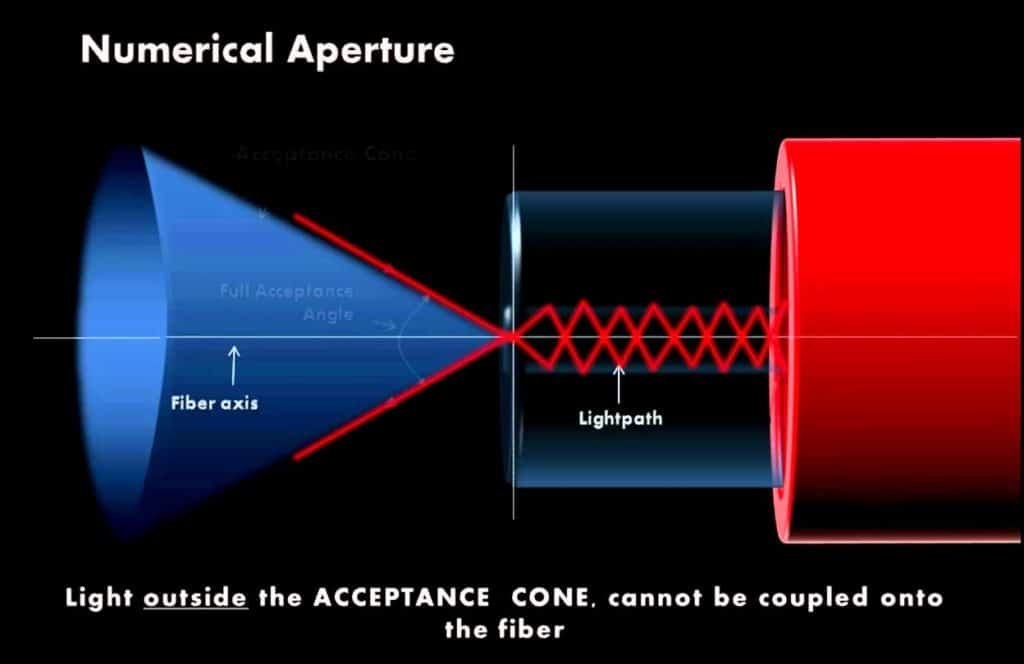# Optical Fiber Communication Basics – Ray theory of light

You might remember studying optics in school. If not, you must remember the experiment where you used a prism to make multicolored light. That phenomenon of splitting of white light into its constituents is known as dispersion. The concepts of reflection and refraction of light are based on a theory known as Ray theory or geometric optics, where light waves are considered as waves and represented with simple geometric lines or rays.

Please note that light has both wave and particle characteristics. Hence, a more accurate portrayal of its behavior is possible by a combination of electromagnetic and quantum theories. However, that does not mean that ray theory is obsolete. Despite its insufficient explanation of many properties of light, it is, in fact, a fairly accurate tool for studying simple phenomena like reflection and refraction especially when the wavelength of light is smaller than the objects it is interacting with.

## Basic laws of ray theory/geometric optics

The basic laws of ray theory are quite self-explanatory

• In a homogeneous medium, light rays are straight lines.
• Light may be absorbed or reflected
• Reflected ray lies in the plane of incidence and angle of incidence will be equal to the angle of reflection.
• At the boundary between two media of different refractive indices, the refracted ray will lie in the plane of incidence. Snell’s Law will give the relationship between the angles of incidence and refraction.

## Reflection of lightThe Law of Reflection states that the angle of incidence must be equal to the angle of reflection.

Reflection depends on the type of surface on which light is incident. An essential condition for reflection to occur with glossy surfaces is that the angle made by the incident ray of light with the normal at the point of contact should be equal to the angle of reflection with that normal.

The images produced from this reflection have different properties according to the shape of the surface. For example, for a flat mirror, the image produced is upright, has the same size as that of the object and is equally distanced from the surface of the mirror as the real object. However, the properties of a parabolic mirror are different and so on.The image has the same size as that of the object, is upright and in the same plane for a flat mirror.

Reflection is also possible in one other scenario even if the surface is not reflective.

## Refraction of lightRefraction is the bending of light in a particular medium due to the speed of light in that medium. The speed of light in any medium can be given by$v=\frac{c}{n}$

Here n is the refractive index of that medium. When a ray of light is incident at the interface of two media with different refractive indices, it will bend either towards or away from the normal depending on the refractive indices of the media.

According to Snell’s law, refraction can be represented as$n_{1}\sin(\theta_{1}) = n_{2}\sin(\theta_{2})$$n_{1}$ = refractive index of first medium$\theta_{1}$ = angle of incidence$n_{2}$ = refractive index of second medium$\theta_{2}$ = angle of refraction

For${ n }_{ 1 }>{ n }_{ 2 }$,$\theta_{2}$ is always greater than$\theta_{1}$. Or to put it in different words, light moving from a medium of high refractive index (glass) to a medium of lower refractive index (air) will move away from the normal.

We made this video to elaborate on Geometric Optics and refraction and explained them using three of the most common applications of refraction that we witness in our daily lives. Check it out!

## Critical Angle

When the angle of refraction is 90 degree to the normal the refracted ray is parallel to the interface between the two media. In this case, the incident angle is called as the critical angle. You can calculate the critical angle using the following formula${ \theta }_{ c }=\sin ^{ -1 }{ \frac { { n }_{ 2 } }{ { n }_{ 1 } } }$It is important to know about this property because reflection is also possible even if the surfaces are not reflective. If the angle of incidence is greater than the critical angle for a given setting, the resulting type of reflection is called Total Internal Reflection, and it is the basis of Optical Fiber Communication.

## Acceptance angle

In an optical fiber, a light ray undergoes its first refraction at the air-core interface. The angle at which this refraction occurs is crucial because this particular angle will dictate whether the subsequent internal reflections will follow the principle of Total Internal Reflection. This angle, at which the light ray first encounters the core of an optical fiber is called Acceptance angle.The objective is to have $\theta_{c}$ greater than the critical angle for this particular setting.As you can notice,$\theta_{c}$ depends on the orientation of the refracted ray at the input of the optical fiber. This in turn depends on$\theta_{a}$, the acceptance angle.

The acceptance angle can be calculated with the help of the formula below.${ \theta }_{ a }=\sin ^{ -1 }{ \left( \frac { { n }_{ 1 } }{ { n }_{ a } } \sin { \left( \frac { \pi }{ 2 } -{ \theta }_{ c } \right) } \right) }$

## Numerical Aperture

Numerical Aperture is a characteristic of any optical system. For example, photo-detector, optical fiber, lenses etc. are all optical systems. Numerical aperture is the ability of the optical system to collect all of the light incident on it, in one area.The blue cone is known as the cone of acceptance. As you can see it is dependent on the Acceptance Angle of the optical fiber. Light waves within the acceptance cone can be collected in a small area which can then be sent into the optical fiber (Source)

The numerical aperture of an optical fiber can be calculated with the following two formulas$NA={ n }_{ 0 }\sin { { \theta }_{ a } }$$NA=\sqrt { { n }_{ 1 }^{ 2 }-{ n }_{ 2 }^{ 2 } }$

These are some basic principles that you should know before we proceed further. You don’t need to know all the formulas just yet. The purpose of this article was to help you revisit these basic concepts from the Ray theory of light.

This site uses Akismet to reduce spam. Learn how your comment data is processed.